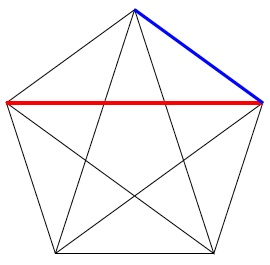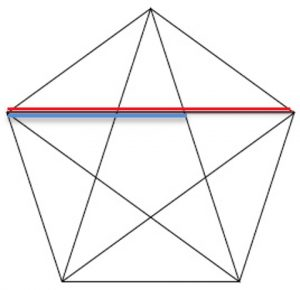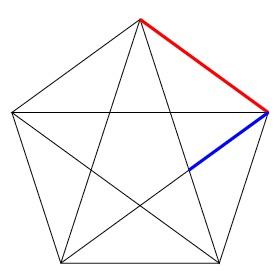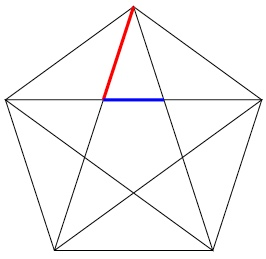Find all the angles in the diagram and mark each set of equal angles in a colour for that set.

Let AE = 1 unit and BE = x units.

Which triangles are isosceles?

Which triangles are similar?

Use similar triangles to give an equation for x and solve the equation.

In each of these diagrams of a regular pentagon find the ratio of the length shown in red to the length in blue in terms of the Golden Ratio $\phi = \frac{1}{2}(1 + \sqrt 5)$.

Prove (1) $\frac{BE}{AE}=\phi$.        (2) $\frac{BE}{BR}=\phi$.            (3) $\frac{AE}{AR}=\phi$.           (4) $\frac{AP}{PR}=\phi$.Tagged with: